### Recent tutorials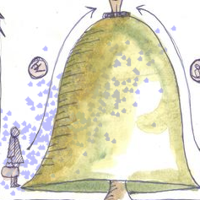#### Generating Multivariate Gaussian Random Numbers

Given a covariance matrix and a mean vector, how do we generate random vectors from the corresponding Gaussian model?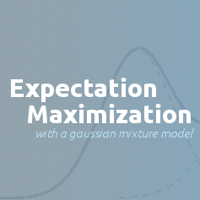#### Expectation Maximization with Gaussian Mixture Models

Learn how to model multivariate data with a Gaussian Mixture Model. For training this model, we use a technique called Expectation Maximization.#### Finding dominant colors in an image

Here's a simple task - given an image find the dominant colors in the image. I'll walk you through a lesser known technique that does not use kmeans.#### Scanning QR Codes

Recognize QR Codes in images from scratch. We'll do all the bit math to figure out the location markers and then read data from the black/white array.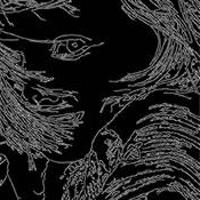#### The Canny Edge Detector

An in-depth exploration of how the famous Canny edge detection system works. We'll implement our own after going through the theory.#### Image Moments

Image moments help identify certain key characteristics in images - like the center, area of white pixels, etc. We'll look at how these are calculated mathematically.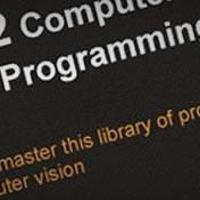#### The OpenCV 2 Computer Vision Application Programming Cookbook

This is a something that their thing is the thing ahalsk the computer vision application programming cookbook and the thing over here is weird.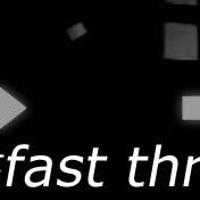#### A super fast thresholding technique

Learn how to implement really fast thresholding - faster than OpenCV! This technique can be a useful addition to your arsenal of computer vision.